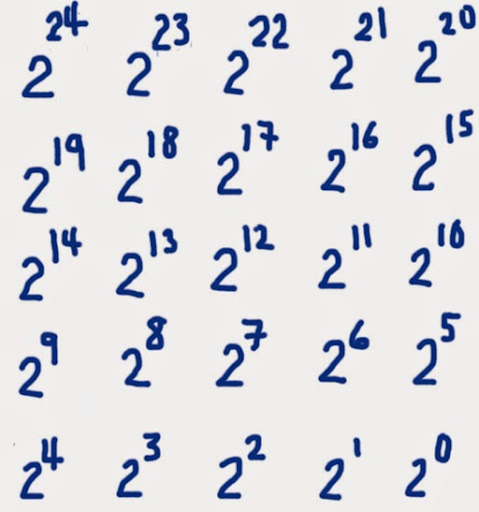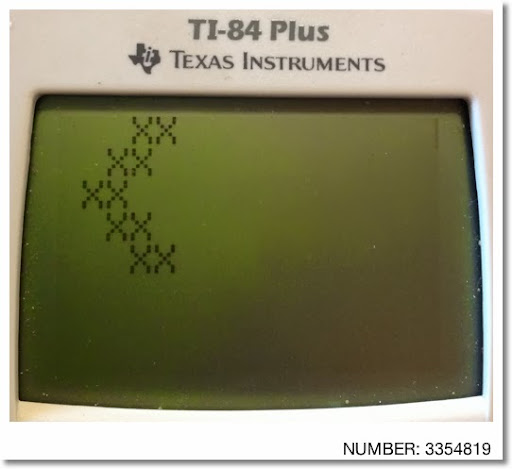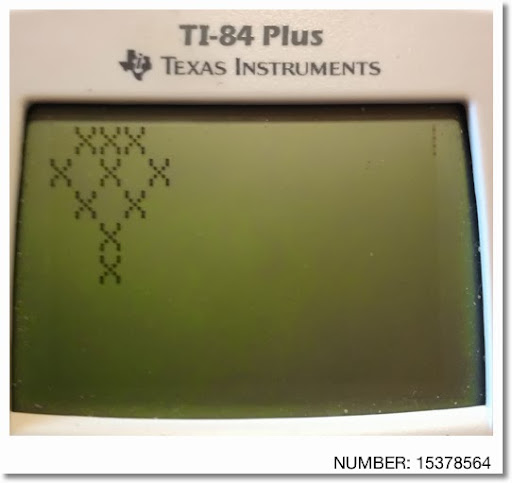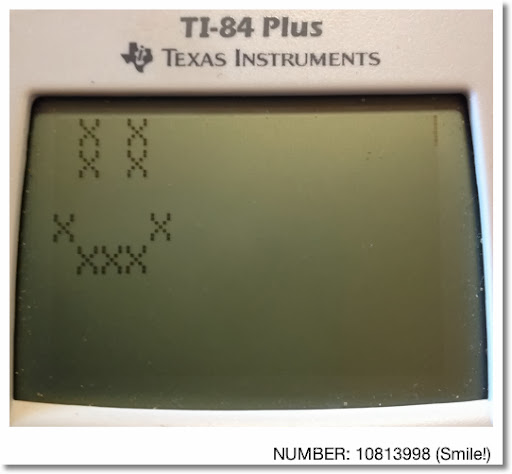## Sunday, January 26, 2014

### TI-84+: BIN2DEC, DEC2BIN, DRAWICON

TI-84+: BIN2DEC, DEC2BIN, DRAWICON

Switching gears for a bit, this blog focuses on the TI-84+:

BIN2DEC: Binary to Decimal: Now allows for any size (up to memory limit).
Input: A list of bits (zeroes and ones). Power of 2 is in descending order from 2^(D-1) to 2^0 where D is the length of the list.

L1: List 1 (2nd, 1; shown as big L with 1 as a subscript)

Example: 11011_2 → 27_10
Input (L1): {1,1,0,1,1}
Output (N): 27

PROGRAM:BIN2DEC
: Input "L1 OF 0 AND 1S:",L1
: dim(L1)→D
: 0→N
: For(K,0,D-1)
: N+2^K*L1(D-K)→N
: End
: Disp "N=DEC"
: Disp N

DEC2BIN: Decimal to Binary
Input: Integer (anything after the decimal point is ignored)
Output: List of binary bits. Power of 2 is in descending order from 2^(D-1) to 2^0 where D is the length of the list.

Example: 5427_10 → 1010100110011_2
Input (N): 5427
Output (L1): {1,0,1,0,1,0,0,1,1,0,0,1,1}

PROGRAM:DEC2BIN
: Input "N:", N
: If N<0
: Then
: Disp "INVALID"
: Stop
: End
: iPart(N)→M
: iPart(ln(M)/ln(2))+1→D
: DelVar L1
: D→dim(L1)
: For(K,0,D-1)
: If 2^(D-1-K)≤M
: Then
: 1→L1(K+1)
: M-2^(D-1-K)→M
: End
: End
: Disp "L1=BIN"
: Pause L1

DRAWICON: Draws a 5 × 5 pixelated pictures using an integer from 1 to 33,554,431. The number is converted into its binary bits. The upper left hand corner represents 2^24. The exponent decreases going right then down each row, with 2^0 representing the bottom right hand corner.

Matrix:PROGRAM:DRAWICON
: 24→N
: Input "NUMBER < 2^25:", M
: If M>2^25-1
: Then
: Disp "EXCESS"
: Stop
: End
: iPart(M)→M
: ClrHome
: For(R,1,5)
: For(C,1,5)
: If 2^N≤M
: Then
: Output(R,C,"X")
: M-2^N→M
: End
: N-1→N
: End
: End
: Pause
: ClrHome

Below are a few examples:Enjoy, and don't forget to make each day count! Until next time,

Eddie

This blog is property of Edward Shore. 2014

### Casio fx-CG50: Sparse Matrix Builder

Casio fx-CG50: Sparse Matrix Builder Introduction The programs can create a sparse matrix, a matrix where most of the entries have zero valu...